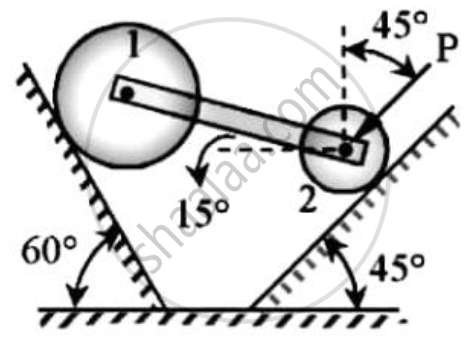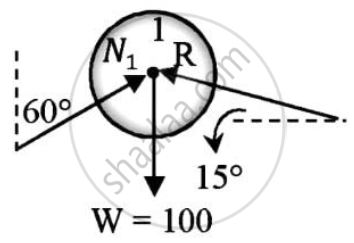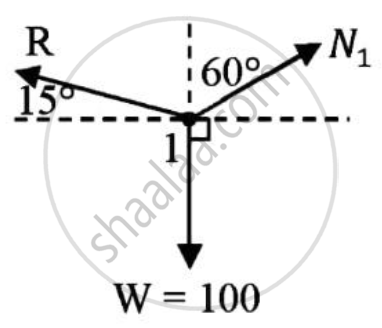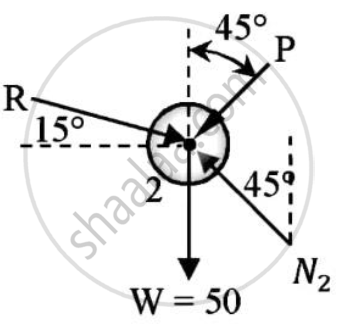# Two Cylinders 1 and 2 Are Connected by a Rigid Bar of Negligible Weight Hinged to Each Cylinder and Left to Rest in Equilibrium in the Position Shown Under the Application of Force P - Engineering Mechanics

Two cylinders 1 and 2 are connected by a rigid bar of negligible weight hinged to each cylinder and left to rest in equilibrium in the position shown under the application of force P applied at the center of cylinder 2.
Determine the magnitude of force P.If the weights of the cylinders 1 and 2 are 100N and 50 N respectively.#### Solution

Given : W1 = 100 N
W2 = 50 N
Cylinders are connected by a rigid bar
To find : Magnitude of force P
Solution :
Consider cylinder IApplying Lami’s theorem :

R/(sin(90+30))=W/sin(60+75)=N_1/sin(90+15)
R=(100)/(sin135)Xsin120
R = 122.4745 N
Cylinder 2 is under equilibriumApplying conditions of equilibrium
ΣFy = 0
N2sin45 - Rsin15 - Psin45 – W = 0
N2sin45 - Psin45 = 122.4745 x 0.2588 + 50
N2sin45 - Psin45 = 81.6987 ………..(1)
Applying conditions of equilibrium
ΣFx = 0
-N2cos45+Rcos15-Pcos45=0
N2cos45+Pcos 45=118.3013 ……(2)
Solving (1) and (2)
P=25.8819 N
Magnitude of force P required = 25.8819 N

Concept: Applications on Equilibrium Mechanisms
Is there an error in this question or solution?